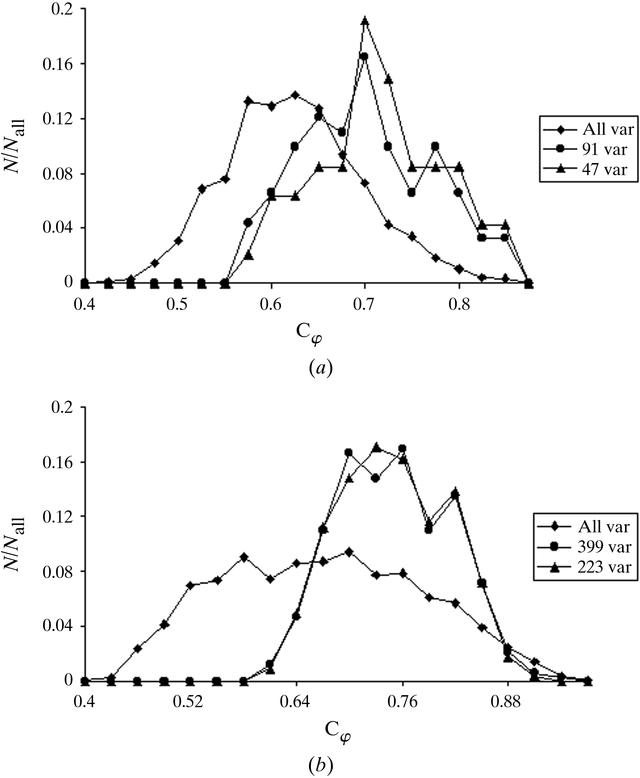disable zoom     view article Figure 4 Comparison of the distributions of Cφ values for all variants randomly generated and for selected variants with maximal GL values. (a) γ-crystallin IIIb; 2000 phase sets were randomly generated at d = 23 Å. The distributions of Cφ are presented for three cases: all 2000 variants, 91 variants with GL ≥ 0.47 and 47 variants with GL ≥ 0.5. The number of pseudo-atoms was N = 100. The parameter Bglob = 100. GL was calculated at d = 20 Å. (b) Elongation factor G; 2000 phase sets were randomly generated at d = 36 Å. The distributions of Cφ are presented for three cases: all 2000 variants, 399 variants with GL ≥ 0.75 and 233 variants with GL ≥ 0.57. The number of pseudo-atoms was N = 100. The parameter Bglob = 4000. GL was calculated at d = 31 Å.BIOLOGICALCRYSTALLOGRAPHY
ISSN: 1399-0047
Volume 56| Part 10| October 2000| Pages 1245-1252